﻿ Dynamical Model for Deriving 1-Min Rain Rate from Various Integration Times in a Tropical Region
Journal of Telecommunications System & Management
• Research Article
• J Telecommun Syst Manage 2016, Vol 5(1): 127
• DOI: 10.4172/2167-0919.1000127

# Dynamical Model for Deriving 1-Min Rain Rate from Various Integration Times in a Tropical Region

Department of Physics, Federal University of Technology, Akure, Nigeria
*Corresponding Author: Ojo JS, Department of Physics, Federal University of Technology, Akure, Nigeria, Tel: 08036710445, Email: [email protected]

Received Date: Aug 28, 2015 / Accepted Date: Dec 16, 2015 / Published Date: Jan 27, 2016

### Abstract

The congestion at the lower frequency bands has forced the telecommunication system engineers to move to higher frequencies. However, microwave signal tends to undergo fading effect at frequencies above 10 GHz due to rainfall. Consequently, the 1-min rain rate data needed for the prediction of rain-induced attenuation is still very scarce in most part of the world, Nigeria inclusive. Therefore, appropriate conversion method is needed to convert the available data to the required 1-min rain rate data. In this paper, an empirical and dynamic method for conversion of long integration time to 1-min integration time has been proposed and compared with other conversion methods. In overall, conversion factor modeled with a power law (CF-PL) is found suitable to be used in this region based on an average rms error less than 2%.

Keywords: Rain rate; Microwave; Attenuation; Integration time; 1-min; Regression coefficient; Tropical location

#### Introduction

Nowadays, operation of wireless communications technology above frequency band of 10 GHz is very important for several daily activities. Frequency band above 10 GHz has several advantages which are able to fulfill the requirements such as wider spectrum availability, high data transfer rate and smaller antenna size . These advantages can provide enough criteria for the development of telecommunication system. However, rain attenuation due to raindrop tend to increase the system noise and causes system lost. As frequency increases, the wavelength of electromagnetic signal reduces to almost the size of rain droplet when the speed is constant. Rain drop affect the propagation of signal in three ways: attenuate the signal by absorbing and scattering the signal, change the signal polarity due to the non-uniform shape of the droplet and increase the system noise temperature which will directly affect the communication links performance . In order to solve the scenario of fading effect, satellite engineer had to design with the least system lost from the prediction of rain attenuation based on 1-min rain rate. According to International Telecommunication Union (ITU), 1-min rain rate is determined to be the best integration time for the prediction of rain induced attenuation . Unfortunately, 1-min rain rate is seldom available whereas rain rate in higher integration time is available in long term statistics in most of the regions. Therefore, suitable conversion method is needed to convert the available rain rate of higher integration time to 1-min integration time.

Several rainfall rate conversion methods applicable in some temperate and tropical countries have been proposed, for example Tattelman and Schar  developed a method for estimating oneminute rainfall rates using monthly mean temperature, monthly mean precipitation, and number of days in the month with precipitation, and latitude. Karasawa and Matsudo  also developed a relationship between one-minute rain rate and one-hour rain rate for 0.01% of time using one-hour rain rate data obtained by Automated Meteorological Data Acquisition System in Japan. One-minute rain rate contour maps for microwave applications in Malaysia Peninsula was also developed by Chebil and Raihman  using 60-min rain rate data. Over the Amritsar region of India, an effort was made by Sharma et al.  to develop a formula for converting 10 min average and 5 min average to equivalent 1 min average rain rate. In the recent study carried out by Selamat et al.  using hydrological data from five areas in East Malaysia, five conversion models were used to convert 60-min rainfall rate to 1-min rainfall rate. In Nigeria, Ajayi and Ofoche  developed a model for converting 5 minutes to 1 minute integration time based on 28-month rain rate data obtained at Ile-Ife, Nigeria. The application of these methods does not however always give good results in areas where the climatic conditions differ from ones in the localities which meteorological data was used when the methods have been developed.

In this paper, an empirical and dynamic model for converting long integration time to 1-min for a tropical location (Akure) has been presented. Several conversion methods were also tested and a suitable model is proposed for this kind of region. An integration time of 10 seconds was averaged over intervals of 1, 5, 10, 30, and 60 minutes and comparison with 1-min rain rate measured in the location was also carried out.

#### Methodology

This section discusses some existing models adopted in this work.

Conversion methods

Power law (PL) model: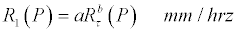(1)

Where R1 (P) and Rτ (P)=1-min and τ-min rainfall rate, τ-min=integration time at which rain rate is available, P=Percentage of exceedance, a and b = Regression coefficients or converting coefficients .

Exponential model: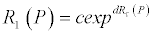(2)

where R1 (P), Rτ (P) and τ remain as defined for equation (1), while c and d are also regression coefficients

Logarithm model: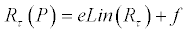(3)

where R1 (P), Rτ (P), τ remain as defined for equation (1), while e and f are also regression coefficients.

Polynomial model:(4)

where R1 (P), Rτ (P), τ remain as defined for equation (1), while g, h and i are also regression coefficients.

Conversion factor modeled with a power law (CF-PL): Another common empirical approach used by Segal  for the conversion of distribution, is the use of a conversion factor, defined as ratio of equiprobable rain rates obtained from each distribution. The conversion factor is then modeled as a function of P, the percentage of time the rain rate is exceeded using power law.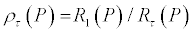(5)

With the conversion factor (P) τ ρ , in the relationship as simple power law: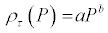(6)

Combining eq (1) and eq (6) gives: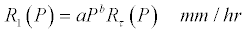(7)

where R1 (P) and Rτ (P)=1-min and τ-min rainfall rate, τ-min= integration time at which rain rate is available, P=Percentage of exceedance, (P) τ ρ =conversion factor at τ-min, a and b=Regression coefficients or converting coefficients, which are obtained through regression. This method is also known as Segal method.

Lavergnat-Gole rainfall statistics conversion method (LG): The LG model Lavergnat et al.  was developed starting from a physical basis: by modeling the time interval separating two consecutive rain drops as a renewal process. The conversion of distributions from integration time τ to the target time of one minute is a specific case of general model and it is achieved by mean of an integration time independent conversion factor defined as the ratio between the two integration times (in this case one minute and τ-minute), as shown in equation 8: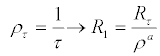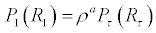(8)

#### Model Testing and Validation

One of the main objectives of this work is to evaluate the performance of various existing conversion models; a test procedure has to be defined. The process of testing and comparison involves calculating, using all selected models, an equivalent P(R)1 using all available P(R)τdistributions per site . The performance metrics selected for the evaluation of the above methods is the relative error ετ, defined in equation (9).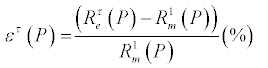(9)

In equation (9) the superscript τ refers to the source integration time, P refers to the percentage of time the rain rate was exceeded and the e and m subscripts represent, respectively, the predicted and measured one minute rain rate values. It is necessary to note that the error variable in equation (9) yields higher errors in the low rain rate portions of the P(R).

In order to select the model that performs best, the root mean square (rms) values of the error variable in equation (6) were calculated. The expressions used are shown in equations (10) to (12) and the result in Table 1.

Models 5- min to 1-min (rms) 15- min to 1-min (rms) 30-min to 1-min (rms) 60-min to 1-min (rms) Average (rms)
PL 0.19 0.61 0.83 1.29 0.73
CF-PL 0.14 0.33 0.67 1.42 0.64
POLY 0.19 0.63 1.08 1.98 0.97
LOG 1.46 1.96 2.09 2.81 2.08
EXP 1.26 1.58 2.00 2.35 1.80
LG 2.79 2.47 2.27 3.69 2.81

Table 1: Performance of prediction models with respect to integration time.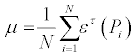(10)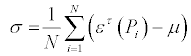(11)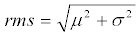(12)

where N denotes the number of error samples available, ετ is the relative error and Pi is the percentage of exceedance. The optimum prediction method is selected on the basis of the magnitude of the rms: the best method would ideally deliver the smallest value of the statistical metrics.

#### Measurements

The measurement site is at Department of Physics, the Federal University of Technology, Akure (FUTA), Nigeria. The 3-year rain rate data collected at Akure (7.17 oN, 5.18 oE) between January 2011 and December, 2013 is used. The measurement was collected using an electronic weather station (Davis 6250 Vantage Vue). The precipitation data was collected using a self-emptying tipping spoon (with a resolution of 0.2 mm per tip), which is part of the Integrated Sensor Suit (ISS). The accuracy of the gauge is about +1% at 1 litter/h with a measuring range of a minimum of 2 mm/h to a 400 mm/h. The instrument scans at an interval of one second and integrated over 1 minute. The availability of the gauge is about 99.2%, the remaining 0.8% unavailability is as a result of system maintenance. Rain rate of other integration times (5-min, 15-min, 30-min and 60-min) have been generated from the self-emptying tipping spoon data. Detailed characteristics of the set up and other information pertaining to the instrument are also available in the work of Ojo et al. .

#### Results and Discussion

The raw rainfall data were converted into rain rate and is classified into several classes based on the rain drop sizes. The rain rate measured 1-min, 5-min, 15-min, 30-min and 60-min integration time distribution is then plotted. Figure 1 shows the measured rain rate distribution for various integration times. The result shows that as the integration time increases, the rain rate is reduced. The rain rate distribution is a function of the rain gauge sampling interval because the intensity of rain is neither spatially nor temporally constant during an event . A precipitation measuring system having a relatively long integration time will always fail to record the short term peaks in rain intensity and thus will tend to underestimate the rain rate at least in the moderate and high intensity region.

Figures 2-7 also show the converted 1-min rain rate data from higher integration time using several conversion methods (CF-PL, PL,Polynomial, Exponential, Logarithm and LG respectively). Referred to four conversions result as shown below, it is obvious that the CFPL, PL and the Polynomial method produced the converted 1-min rain rate closest to the measured 1-min rain rate for 5-min integration time at equa1 percentage of exceedance. For 15-min and 30-min integration time almost all the methods have equal deviation from the 1-min measured rain rate. For 60-min integration time the deviation is higher than other smaller integration times at the same percentage of exceedance.

The deviation from the 1-min measured rain rate increases as integration time increases for all the conversion methods, except the for the LG method in which the 30-min integration time deviate more than the 60-min integration time. This may be as a result of the nature of the conversion method which is semi-empirical i.e physical-stochastic in nature, making use of one parameter a for the conversion process.

Performance of different models compared to 1-min measured

Figures 2-7 also show the converted 1-min rain rate data from higher integration time using several conversion methods. From the results it is evident that CF-PL, PL and Polynomial method produced the converted 1-min rain rate closest to the 1-min measured rain rate at equa1 percentages of exceedance.

For 15-min and 30-min integration time almost all the methods have equal deviation from the 1-min measured rain rate. For 60-min integration time the deviation is higher than other smaller integration times at the same percentages of exceedance.

The deviation from the 1-min measured rain rate increases as integration time increases for all the conversion methods, except for the LG method in which the 15-min and 30-min integration time deviate more than the 5-min integration time. This may be as a result of the nature of the conversion method which is semi-empirical method, making use of one parameter for the conversion process.

Figure 8 and Table 1 show the results of the model testing as a function of integration times. Results obtained presented in the figure and the table based on equations (10) to (12) show that the error variable yields higher errors in the high integration time portions.

Tables 2-4 also present the comparison of the present results with regional results, global results and general ranking of the models respectively. The regional and global results were obtained from the work of Emiliani et al. . It could be observed that the Lavergnat- Gole model is not prone to errors and performs best overall integration times and over all the climatic regions, with small changes in the overall rms of the conversion error.

Models Tropical climate region (rms) Temperate climate region (rms) Cold climate region (rms) Present work (Tropical climate). (rms)
PL 21.08 12.33 18.09 0.73
CF-PL 21.08 11.31 15.49 0.64
LG 16.38 12.77 15.04 2.08

Table 2: Comparison with Regional results.

 Models Global Present work 5 to 1-min 30  to 1-min 60  to 1-min 5 to 1-min 30 to 1-min 60 to 1-min (rms) (rms) (rms) (rms) (rms) (rms) PL 6.70 23.67 31.82 0.19 0.83 1.29 CF-PL 9.53 19.61 30.25 0.14 0.67 1.42 LG 10.30 17.99 22.04 1.46 2.81 2.09

Table 3: Comparison with global results.

Models Global Tropical climate Temperate climate Cold climate Present climate
PL 3 3 1 2 2
CF-PL 2 2 2 3 1
LG 1 1 3 1 3

Table 4: Ranking of models, based on global, regional and present work rms values reported in Tables 2 and 3.

#### Conclusion

The rainfall data collected at Akure, south western Nigeria between 2011 to 2013 have been utilized to study the effect of integration time on cumulative distribution of rain rate for a tropical station. Values of equiprobable rain rate for different integration times for probabilities between the range of 0.001% and 1% have been employed to obtain a power law relationship and other relations between the rain rates at different integration times.

The overall results show that the rain rate converted using CFPL method provided the best estimator with the lowest error rate on the average for the 1-minute rainfall rate distribution in Akure. This method can be used effectively to estimate the 1-minute rainfall rate distribution for tropical locations in the South West Nigeria.

The ranking of the models performance based on the present study, regional and global results suggest that the Lavergnat-Gole model is not prone to errors and performs best overall integration times and over all the climatic regions, with small changes in the overall rms of the conversion error. The review also showed how empirical methodologies represent an alternative due to their simplicity. However, it also highlighted the dependency of the performance of empirical methodologies on the integration time with rms increases with increasing integration times. In overall, conversion factor modeled with a power law (CF-PL) is found suitable to be used in this region based on an average rms error less than 2%.

#### References

Citation: Ojo JS, Adenugba AK, Adediji AT (2016) Dynamical Model for Deriving 1-Min Rain Rate from Various Integration Times in a Tropical Region. J Telecommun Syst Manage 5: 127. Doi: 10.4172/2167-0919.1000127

Copyright: ©2016 Ojo JS, et al. This is an open-access article distributed under the terms of the Creative Commons Attribution License, which permits unrestricted use, distribution, and reproduction in any medium, provided the original author and source are credited.

Select your language of interest to view the total content in your interested language

##### Recommended Journals
Viewmore
###### Article Usage
• Total views: 9613
• [From(publication date): 3-2016 - Dec 13, 2019]
• Breakdown by view type
• HTML page views: 9429Can't read the image? click here to refresh